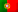Home > Technology > Electrical engineering > Resistors in series and parallel circuits

# Resistors in series and parallel circuits

### Introduction

In the area of electrical engineering, one of the basic subjects we learn are circuits of direct current with resistors in series and in parallel. To start with, let’s remind that in a basic DC circuit we have a voltage source, a total resistance and a electrical current.

Let’s also remind the Ohm’s law: V = R.I

Where

• V (or U) = Voltage (or electrical tension) measured in volts (V)
• R = Electrical resistance measured in ohms (Ω)
• I = Intensity of the electrical current measured in amperes (A)

When we have circuits with several resistors in series, parallel, or series and parallel, it’s important to know how to determine the total resistance (or equivalent resistance), so we can determine other measurements like voltage or electrical current.

### Resistors in series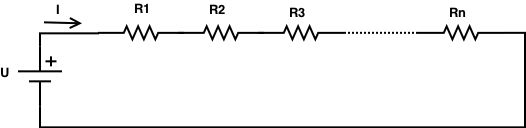Let’s then start with a series circuit with resistors. In the figure above we have “n” resistors with the values R1, R2, R3, … and Rn. To determine the equivalent resistance, we sum all the resistances of each resistor:

\$\$R_{eq} = ∑↙{i=1}↖n R_i = R_1 + R_2 + … + R_{n-1} + R_n (Ω)\$\$

So, for instance, if we have a series circuit with 3 resistors: R1 = 8Ω ; R2 = 4Ω and R3 = 10Ω we will have an equivalent resistance of:

\$\$R_{eq} = R_1 + R_2 + R_3 = 8 + 4 + 10 = 22Ω\$\$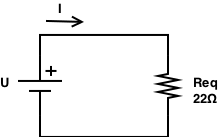This means we could have another circuit with just one resistor (Req) of 22Ω, as shown in the picture on the right.

Now, it will be easier to determine other measurements. Let’s say we have this circuit connected to a voltage source of 44V and we want to determine the value of the electrical current. We can simply apply the Ohm’s law:

\$\$V=R.I⇔I = V/R = 44/22 = 2A\$\$

In a series circuit the current that flows through each resistor is always the same. If we have “n” resistors, we will have:

\$\$I = I_1 = I_2 = I_3 = … = I_{n-1} = I_n\$\$

Regarding the voltage applied in the circuit, it will be equal to the sum of the voltage drop in each resistor:

\$\$V = ∑↙{i=1}↖n V_i = V_1 + V_2 + … + V_{n-1} + V_n (V)\$\$

This means that, in each resistor we will have a voltage drop that will be equal to the resistance of that resistor times the current that flows through it, and for series circuits we know that this current is always the same and with a value of I.

If we consider the previous example, we will have the following voltage drops:

• V1 = R1 × I = 8 × 2 = 16V
• V2 = R2 × I = 4 × 2 = 8V
• V3 = R3 × I = 10 × 2 = 20V

If we sum all the voltage drops, we will get: 16 + 8 + 20 = 44V!

(Note that is also very common to represent a voltage drop as ΔV, so in this case we would have ΔV1, ΔV2 and ΔV3)

### Resistors in parallel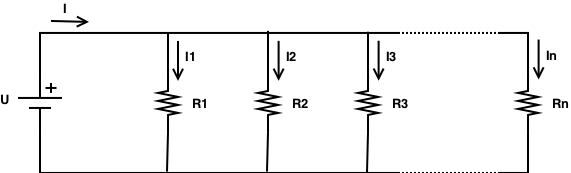When we have a parallel circuit with resistors, the calculations will be quite different and the equivalent resistance (or total resistance) will be given by:

\$\$1 / R_{eq} = ∑↙{i=1}↖n 1/ R_i =1/ R_1 +1/ R_2 + … + 1/R_{n-1} + 1/R_n (Ω)\$\$

This means that, the reciprocal of the equivalent resistance is equal to the sum of the reciprocal of each resistance.

Let’s now consider we have a circuit with 3 resistors in parallel and with the same values of those we used in the series circuit. So, we have: R1 = 8Ω ; R2 = 4Ω e R3 = 10Ω

The equivalent resistance will be:

\$\$1/R_{eq}=1/8 + 1/4 + 1/10 ⇔ 1/R_{eq}=19/40 ⇔ R_{eq} = 40/19 ≈2.1Ω\$\$

We can see here that the total resistance is lower than the value of the lowest resistance in the circuit!

If we keep the same voltage source we used in the example of the series circuit, of 44V, notice that now the value of the electrical current, for this parallel circuit, will be:

\$\$V=R.I⇔I = V/R = 44/2.1 ≈ 20.9A\$\$

Comparing with the current in the series circuit, this is more than 10X higher! This fact can be explained if we remind that, when we have a series circuit, the total resistance will be the sum of all resistors and, in the parallel circuit, we have that the reciprocal of the total resistance is given by the sum of the reciprocal of each resistor. Thus, leading to a much lower value.

As we kept the same voltage source, we know that the lower the total resistance is, the higher the current will be! Let’s also see how the currents and the voltages will behave in a parallel circuit.

– The total electrical current in the circuit is equal to the sum of all currents that flow through each “branch” of resistances. In the circuit above, it is possible to see I1, I2, I3, … and In. In fact, we have:

\$\$I= ∑↙{i=1}↖n I_i = I_1 + I_2 + … + I_{n-1} + I_n (A)\$\$

– The voltage applied in the circuit is always equal to the voltage drop in each “branch” of the parallel. This is:

\$\$V=V_1 = V_2 = … = V_{n-1} = V_n\$\$

So, in this parallel circuit example, the voltage drop in all the resistors is the same and it is 44V.

Regarding the electrical currents, we will have:

\$\$I_1 = U / R_1 = 44 / 8 = 5.5A\$\$

\$\$I_2 = U / R_2 = 44 / 4 = 11A\$\$

\$\$I_3 = U / R_3 = 44 / 10 = 4.4A\$\$

If we sum all the values of these currents, we will get the total value of 20.9A.

### Putting all together with a resolved exercise

Let’s consider the circuit presented in the beginning of this post, but now with some values on it:

• R1 = 10Ω; R2 = 20Ω; R3 = 30Ω; R4 = 10Ω e R5 = 25Ω
• I = 5A

We want to know:

• The value of the source voltage V (or U)
• The value of the current I3 (that flows through R3) amd I24 (that flows through R2 and R4)
• The voltage drop U4 in the resistor R4

Let’s redraw the circuit in order to avoid errors and to help us with the calculations: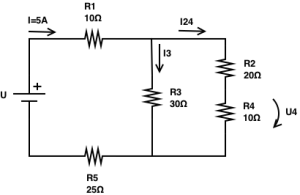So we have a resistor R1 in series with a parallel and also in series with R5. Let’s start with the parallel where we have two “branches”. One with the resistor R3 and another with the resistors R2 and R4 in series.

– Series of R2 and R4:

\$\$R_{24} = R_2 + R_4 = 20Ω + 10Ω = 30Ω\$\$

– Parallel of R3 with R24:

\$\$1/R_{324} = 1/R_3 + 1/R_24 = 1/30 + 1/30 ⇔ 1/R_{324} = 2/30 ⇔ R_{324} = 30/2 = 15Ω\$\$

Notice that, as the values of the resistances in the parallel are the same, the value of the total resistance is half1.

– Finally, we can now determine the total resistance of the circuit:

\$\$R_{eq} = R_1 + R_{324} + R_5 = 10Ω + 15Ω + 25Ω = 50Ω\$\$

– The source voltage will then be given by:

\$\$V = R×I = 50 × 5 = 250V\$\$

– We know that the voltage drop in each resistor of a parallel circuit is the same of the voltage source. But we can’t assume that on this case and say that the voltage drop in the parallel branches will be 250V! Notice that we also have two other resistors in series with the parallel, R1 and R5! So, we need to determine the voltage drops in R1 and R5! To help you to better understand this, let’s now change the position of the resistors in the circuit: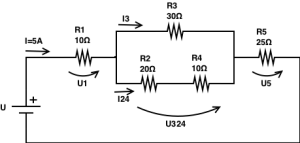You can see that the circuit is the same, but displayed in another way. Ignoring the voltage drop in R4 by now, we can see we have here 3 voltage drops to have into account: V1, V324 (in the parallel) and V5.

\$\$V_1 = R_1 × 5 = 10 × 5 = 50V\$\$

\$\$V_{324} = R_{324} × 5 = 15 × 5 = 75V\$\$

\$\$V_5 = R_5 × 5 = 25 × 5 = 125V\$\$

Verifying the sum of the voltage drops:

\$\$V=V_1 + V_{324} + V_5 = 50V + 75V + 125V = 250V\$\$

Now we know that the voltage drop in the parallel is 75V and not 250V! Therefore, we can now determine the requested electrical currents:

\$\$I_3 = U_{324} / R_3 = 75/30 = 2.5A\$\$

\$\$I_{24} = U_{324} / R_{24} = 75/30 = 2.5A\$\$

If you see, we have the same values for the currents in each “branch”! Is it odd? Not at all! Notice that the resistance is the same in both two “branches”, thus the current will be divided in equal parts2.

Finally, as we now know the value of the electrical current that flows through the resistors R2 and R4 is 2.5A, we can determine the voltage drop in R4:

\$\$V_4 = R_4 × I_{24} = 10 × 2.5 = 25V\$\$

Footnotes:

1. When we have a strict parallel circuit where all the resistances for each “branch” are the same and with a value of R, and considering we have “n” parallel branches, we can simplify the expression to determine the equivalent resistance:
\$\$ R_{eq} = R / n\$\$
2. In the same case, we can verify that the currents will be distributed with equal values for each one of the “n” branches, so we can affirm that, for each branch “i”, the value of the current will always be given by:
\$\$ I_i = I /n\$\$